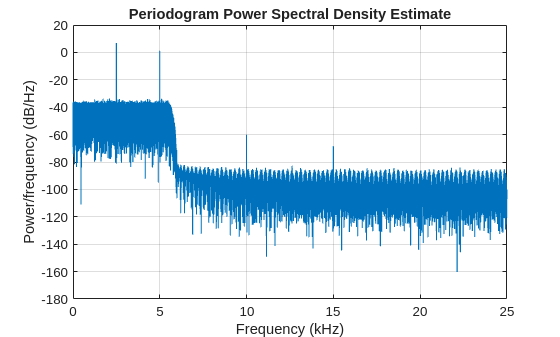fftfilt

FFT-based FIR filtering using overlap-add method

Description

example

y = fftfilt(b,x) filters the data in vector x with the filter described by coefficient vector b.

y = fftfilt(b,x,n) uses n to determine the length of the FFT.

y = fftfilt(d,x) filters the data in vector x with a digitalFilter object d.

y = fftfilt(d,x,n) uses n to determine the length of the FFT.

example

y = fftfilt(gpuArrayb,gpuArrayX,n) filters the data in the gpuArray (Parallel Computing Toolbox) object gpuArrayX with the FIR filter coefficients in the gpuArray stored in gpuArrayb.

Examples

collapse all

Verify that filter is more efficient for smaller operands and fftfilt is more efficient for large operands. Filter ${10}^{6}$ random numbers with two random filters: a short one, with 20 taps, and a long one, with 2000. Use tic and toc to measure the execution times. Repeat the experiment 100 times to improve the statistics.

rng default

N = 100;

shrt = 20;
long = 2000;

tfs = 0;
tls = 0;
tfl = 0;
tll = 0;

for kj = 1:N

x = rand(1,1e6);

bshrt = rand(1,shrt);

tic
sfs = fftfilt(bshrt,x);
tfs = tfs+toc/N;

tic
sls = filter(bshrt,1,x);
tls = tls+toc/N;

blong = rand(1,long);

tic
sfl = fftfilt(blong,x);
tfl = tfl+toc/N;

tic
sll = filter(blong,1,x);
tll = tll+toc/N;

end

Compare and display the average times.

fprintf('%4d-tap filter averages: fftfilt: %f s; filter: %f s\n',shrt,tfs,tls)
20-tap filter averages: fftfilt: 0.176911 s; filter: 0.017986 s
fprintf('%4d-tap filter averages: fftfilt: %f s; filter: %f s\n',long,tfl,tll)
2000-tap filter averages: fftfilt: 0.066474 s; filter: 0.129841 s

This example requires Parallel Computing Toolbox™ software. Refer to GPU Support by Release (Parallel Computing Toolbox) for a list of supported GPUs.

Create a signal consisting of a sum of sine waves in white Gaussian additive noise. The sine wave frequencies are 2.5, 5, 10, and 15 kHz. The sampling frequency is 50 kHz.

Fs = 50e3;
t = 0:1/Fs:10-(1/Fs);
x = cos(2*pi*2500*t) + 0.5*sin(2*pi*5000*t) + 0.25*cos(2*pi*10000*t)+ ...
0.125*sin(2*pi*15000*t) + randn(size(t));

Design a lowpass FIR equiripple filter using designfilt.

d = designfilt('lowpassfir','SampleRate',Fs, ...
'PassbandFrequency',5500,'StopbandFrequency',6000, ...
'PassbandRipple',0.5,'StopbandAttenuation',50);
B = d.Coefficients;

Filter the data on the GPU using the overlap-add method. Put the data on the GPU using gpuArray. Return the output to the MATLAB® workspace using gather and plot the power spectral density estimate of the filtered data.

y = fftfilt(gpuArray(B),gpuArray(x));
periodogram(gather(y),rectwin(length(y)),length(y),50e3)Input Arguments

collapse all

Filter coefficients, specified as a vector. If b is a matrix, fftfilt applies the filter in each column of b to the signal vector x.

Input data, specified as a vector. If x is a matrix, fftfilt filters its columns. If b and x are both matrices with the same number of columns, the ith column of b is used to filter the ith column of x. fftfilt works for both real and complex inputs.

FFT length, specified as a positive integer. By default, fftfilt chooses an FFT length and a data block length that guarantee efficient execution time.

Digital filter, specified as a digitalFilter object. Use designfilt to generate d based on frequency-response specifications.

GPU arrays, specified as a gpuArray object. gpuArrayb contains the filter coefficients, and gpuArrayX is the input data. See Run MATLAB Functions on a GPU (Parallel Computing Toolbox) for details on gpuArray objects. Using fftfilt with gpuArray objects requires Parallel Computing Toolbox™ software. Refer to GPU Support by Release (Parallel Computing Toolbox) for a list of supported GPUs. The filtered data, y, is a gpuArray object. See Overlap-Add Filtering on the GPU for an example of overlap-add filtering on the GPU.

Output Arguments

collapse all

Output data, returned as a vector, matrix or gpuArray object.

collapse all

Comparison to filter function

When the input signal is relatively large, fftfilt is faster than filter.

filter performs N multiplications for each sample in x, where N is the filter length. fftfilt performs 2 FFT operations — the FFT of the signal block of length L plus the inverse FT of the product of the FFTs — at the cost of $\frac{1}{2}L{\mathrm{log}}_{2}L$ where L is the block length. It then performs L point-wise multiplications for a total cost of $L+L{\mathrm{log}}_{2}L=L\left(1+{\mathrm{log}}_{2}L\right)$ multiplications. The cost ratio is therefore $L\left(1+{\mathrm{log}}_{2}L\right)/\left(NL\right)=\left(1+{\mathrm{log}}_{2}L\right)/N$ which is approximately log2L / N.

Therefore, fftfilt is faster when log2L is less than N.

Algorithms

fftfilt filters data using the efficient FFT-based method of overlap-add , a frequency domain filtering technique that works only for FIR filters by combining successive frequency domain filtered blocks of an input sequence. The operation performed by fftfilt is described in the time domain by the difference equation:

$y\left(n\right)=b\left(1\right)x\left(n\right)+b\left(2\right)x\left(n-1\right)+\cdots +b\left(nb+1\right)x\left(n-nb\right)$

An equivalent representation is the Z-transform or frequency domain description:

$Y\left(z\right)=\left(b\left(1\right)+b\left(2\right){z}^{-1}+\cdots +b\left(nb+1\right){z}^{-nb}\right)X\left(z\right)$

fftfilt uses fft to implement the overlap-add method. fftfilt breaks an input sequence x into length L data blocks, where L must be greater than the filter length N.and convolves each block with the filter b by

y = ifft(fft(x(i:i+L-1),nfft).*fft(b,nfft));

where nfft is the FFT length. fftfilt overlaps successive output sections by n-1 points, where n is the length of the filter, and sums them.fftfilt chooses the key parameters L and nfft in different ways, depending on whether you supply an FFT length n for the filter and signal. If you do not specify a value for n (which determines FFT length), fftfilt chooses these key parameters automatically:

• If length(x) is greater than length(b), fftfilt chooses values that minimize the number of blocks times the number of flops per FFT.

• If length(b) is greater than or equal to length(x), fftfilt uses a single FFT of length

2^nextpow2(length(b) + length(x) - 1)

This computes

y = ifft(fft(B,nfft).*fft(X,nfft))

If you supply a value for n, fftfilt chooses an FFT length, nfft, of 2^nextpow2(n) and a data block length of nfft - length(b) + 1. If n is less than length(b), fftfilt sets n to length(b).

 Oppenheim, Alan V., Ronald W. Schafer, and John R. Buck. Discrete-Time Signal Processing. 2nd Ed. Upper Saddle River, NJ: Prentice Hall, 1999.